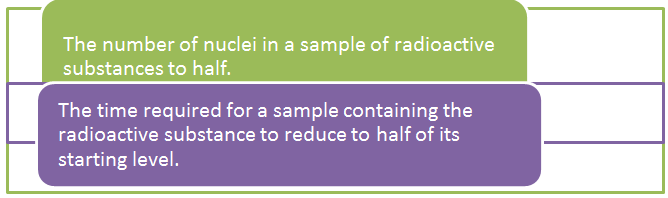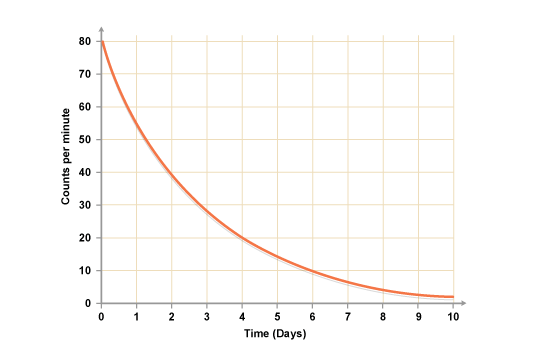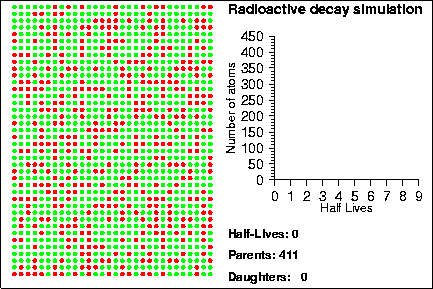×#### Thank you for registering.

One of our academic counsellors will contact you within 1 working day.

Click to Chat

1800-1023-196

+91-120-4616500

CART 0

• 0

MY CART (5)

Use Coupon: CART20 and get 20% off on all online Study Material

ITEM
DETAILS
MRP
DISCOUNT
FINAL PRICE
Total Price: Rs.

There are no items in this cart.
Continue Shopping• Complete Physics Course - Class 11
• OFFERED PRICE: Rs. 2,968
• View Details

```Half-Life

Table of Content

Half-Life is the time taken for

Modern Half-Life formulae

How is half-life useful in carbon dating

Uses of the half-life in NDT

Related Resources

According to the law giving quantitative description about radioactivity, the rate of disintegration decreases with a decrease in the number of atoms, i.e., when the number of atoms left behind is small, rate of disintegration also would have fallen considerably.We know that the nuclei of radioactive substances are unstable. They break down i.e. disintegrate into a completely new atom and this process is termed as radioactive decay. The radioactivity of an object is indeed measured by the number of nuclear decays it emits every second. Hence, the more it emits, the more radioactive the substance is.

The half-life of a radioactive substance, as the name suggests is the time taken for half its radioactive atoms to decay. Half-life of a radioactive substance is defined as the time during which the number of atoms of the substance are reduced to half their original value. It is not generally possible to predict when a particular atom will decay or not. However, with the help of half-life it becomes possible to calculate the time taken by half of the nuclei of radioactive substance to decay. There may be several definitions of half-life, but they basically imply the same thing.

Half-Life is the time taken forThe half-lives of different radioactive substances are different. We list below the half-lives of some of the known sample elements:

Isotope
Half-life

Carbon-14
5,715 years

Francium-223
20 minutes

Oxygen 16
infinite

Uranium 238
4,460,000,000 years

Uranium 235
713,000,000 years

Cobalt 60
5.27 years

Silver 94
42 seconds

The figure given below further illustrates the concept of half-life. It shows the original substance and then what happens to it after it decays post one half-life and two half-lives.Modern Half-life formulae

The graph shows the decay curve for a radioactive substance. It shows the original substance and then what happens to it after it decays post one half-life and two half-lives. Radioactivity decreases with time. It is possible to find out the half-life of a radioactive substance from a graph of the count rate against time.There are various ways of measuring half-life of substances. We give three main formulae to measure half-life:

N (t) = N0 e-t/τ

N (t) = N0 e -λt

Here,

N0 is the initial quantity of the substance that will decay,

N(t) is the quantity that still remains and has not yet decayed after a time t,

t1/2 is the half-life of the decaying quantity,

τ is a positive number which denotes the mean lifetime of the decaying quantity,

λ is a positive number called the decay constant of the decaying quantity.

A significant feature of the above graph is the time in which the number of active nuclei (N) is halved. This is independent of the starting value, N = N0. Let us compute this time:

N0/2 = N0e–λr'

= e–λr' = 1/2

or, λt'  = In 2 = 0.693 (approx)

or,  t' = T1/2  = 0.693/λ

This half-life represents the time in which the number of radioactive nuclei falls to 1/2 of its starting value. Activity, being proportional to the number of active nuclei, also has the same half-life.Life of all the radioactive elements is infinite.

Radioactive decay constant ‘λ’, of different elements different. Elements having greater ‘λ’ have smaller half-life.

Half-life of a radioactive substance is inversely proportional to its radioactive decay.

The radioactivity of an object is measured by the number of nuclear decays it emits each second – the more it emits, the more radioactive it is.

The half-life of radioisotopes varies from seconds to billions of years.

Carbon-dating uses the half-life of Carbon-14 to find the approximate age of an object that is 40,000 years old or younger.

Watch this Video for more reference

How is half-life useful in carbon dating?

The half-lives of radioactive substances facilitate the determination of the ages of very old artifacts. It is quite useful for the scientists like the half-life of Carbon-14 can be used to get an idea about the approximate age of organic objects less than 40000 years old. By finding out the quantity of carbon-14 that has trans mutated, the scientists can estimate a rough idea about the age of a substance. This is called the process of Carbon-dating.

Uses of the half-life in NDT

Problem 1 (JEE Main)

There are two radioactive substances A and B. Decay constant of B is two times that of A. Initially both have equal number of nuclei. After n half lives of A rate of disintegration of both are equal. The value of n is

(a) 1              (b) 2

(c) 4              (d) All of these

Solution:-

Let λA = λ    and λB = 2λ

Initially rate of disintegration of A is λN0 and that of B is 2λN0.

After one half-life of A, rate of disintegration of A will becomes λN0/2 and that of B would also be λN0/2 (half-life of B = ½ half-life of A).

So, after one half-life of A or two half-lives of B.

(– dN/dt)A = (– dN/dt)B

Thus, n = 1

From the above observation we conclude that, option (a) is correct.

Problem 2:-

A count rate-meter is used to measure the activity of a given sample. At one instant the meter shows 4750 counts per minute. Five minutes later it shows 2700 counts per minute. Find:

(a)  Decay constant

(b) The half-life of the sample

Solution:-

We are required to find the decay constant and the half-life of the sample.

So, we know the Initial activity = A0 = dN/dt at t = 0

Final activity = At = dN/dt at t = t

=> 47500 / 2700 = N0/Nt

Using λt = 2.303 log  N0/Nt

=> λ (5) = 2.303 log 4750/2700

=>λ= 2.303 / 5 log 4750/2700 = 0.1129 min–1

Therefore, t1/2 = 0.693 / 0.1129 = 6.14 min

Thus, from the above observation we conclude that, the half life of the sample would  be 6.14 min.Question 1:-

The half life of a radioactive decay is ‘x’ times its mean life. Here x is given by:

(a) 0.3010          (b) 0.6930      (c) 0.5020     (d) 1/0.6930

Question 2:-

The half life of a phosphorus-32 is 15 days. A given mass of phosphorus-32 will be reduced to ¼ th of its original mass in:

(a) 60 days     (b) 30 days    (c) 45 days     (d) 90 days

Question 3:-

What percentage of original radioactive atoms is left after five half lives?

(a) 20%     (b) 10%     (c) 5%    (d) 3%

Question 4:-

The half life of radioactive radon is 3.8 days. The time at the end of which 1/20 th of the radon sample will remain undecayed in (given log10e = 0.4343):

(a) 13.8 days       (b) 16.5 adys

(c) 33 days          (d) 76 days

Question 5:-

Half-life of a radioactive element depends upon:

(a) amount of element present                  (b) temperature

(c) pressure                                            (d) none of the aboveQ.1
Q.2
Q.3
Q.4
Q.5

b

b

d

b

d

Related Resources

You might like to refer Nuclear Fusion.

For getting an idea of the type of questions asked, refer the  Previous Year Question Papers.

```### Course Features

• 101 Video Lectures
• Revision Notes
• Previous Year Papers
• Mind Map
• Study Planner
• NCERT Solutions
• Discussion Forum
• Test paper with Video Solution